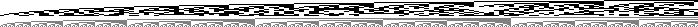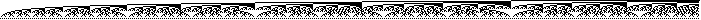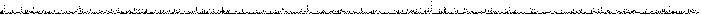Math Games Sequence Pictures Ed Pegg Jr., December 8, 2003

Mike Shafer found a term in sequence A000043 last week. When 2 is raised to the power of a number from {2, 3, 5, 7, 13, 17, 19, 31, 61, 89, 107, 127, 521, 607, 1279, 2203, 2281, 3217, 4253, 4423, 9689, 9941, 11213, 19937, 21701, 23209, 44497, 86243, 110503, 132049, 216091, 756839, 859433, 1257787, 1398269, 2976221, 3021377, 6972593,13466917, 20996011}, and one is subtracted, a prime results. This week, I took an extended look at this and other sequences in OEIS, by converting them into pictures. Here's the picture for Mersenne prime exponents (A000043).Figure 1. Mersenne Prime Exponents (A000043).

I should explain how this picture is made. In binary, 20996011 is {1, 0, 1, 0, 0, 0, 0, 0, 0, 0, 1, 0, 1, 1, 1, 1, 1, 1, 0, 1, 0, 1, 0, 1, 1}. Take a look at the last column in the image, and you'll see that the binary number has been turned into black and white squares, reading down. Underneath that, i've placed binary representations of the natural numbers (1, 2, 3, 4, 5 ...). When I made this picture, I faintly hoped I might see some obvious pattern. I didn't.

Here are the whole numbers / natural numbers / positive integers up to 700, in binary columns.Figure 2. The positive integers (A000027).

How many different ways can n cents be represented with 1, 5, 10, and 25 cent coins?Figure 3. Ways to make change (A001299).

Here are the triangular numbers, square numbers, and cubic numbers.Figure 4. Triangular (A000217), Square (A000290), and Cubic numbers (A000578).

The Gray Code arranges the numbers so that only one binary bit changes at a time. It's used in satellite sensor arrays.Figure 5. The Gray Code (A003188).

From the Small Groups Library, here is the number of groups of order n. Note the spikes at powers of 2.Figure 6. Groups of order n. (A000001).

The numbers in Pascal's Triangle, which begin as 1, 1,1, 1,2,1, 1,3,3,1, 1,4,6,4,1 ... If you like the picture, you might like to hear the sequence, at the Sound of Mathematics page.Figure 7. Pascal's Triangle (A007318).

Other sequence pictures look more chaotic.Figure 8. Continued Fraction of Pi (A001203), order of SL(2,Z_n) (A000056), the Primes (A000040).

The following lists the number of divisors of n. This is also the number of Pythagorean triangles with an inscribed circle of radius n.Figure 9. τ(n): Divisors of n (A000005).

My favorite picture surprised me. It's the Fibonacci sequence. Until I saw it, I didn't occur to me that it would have these internal patterns.Figure 10. The Fibonacci Sequence (A000045).

In Stephen Wolfram's book A New Kind of Science, many sequence pictures can be found in Chapter 4. The powers of 3/2 makes a fantastic picture. Many more can be seen at the Color NKS Images page. As a larger effort, functions.wolfram.com has made available thousands of images that can be generated by functions.

For more on sequences, please see N J A Sloane's paper, My Favorite Integer Sequences.

References:

Sloane, N J A. Sequences A000001, A000005, A000027, A000040, A000043, A000045, A000056, A000217, A000290, A000578, A001203, A001299, A003188, A007318 in "The On-Line Encyclopedia of Integer Sequences." http://www.research.att.com/~njas/sequences/.

Weisstein, E W. Pascal's Triangle Eric Weisstein's World of Mathematics. http://mathworld.wolfram.com/.

Wolfram, S. A New Kind of Science. Champaign, IL: Wolfram Media, 2002.

Mathematica Code:

(*Initialization*) RasterGraphics[state_, colors_:2, size_:1] := With[{dim = Reverse[ Dimensions[state]]}, Graphics[ Raster[ Reverse[1 - state/(colors - 1)]], AspectRatio -> Automatic, PlotRange -> {{0, dim[]}, {0, dim[]}}, ImageSize -> size*dim + 1]]

(*Figure 10*) With[{seq = Table[Fibonacci[n], {n, 1, 700}]}, Show[ RasterGraphics[ Join[2 Transpose[ Map[ IntegerDigits[#,2, Ceiling[ Log[2, Max[seq]]]] &, seq]],Transpose[Table[IntegerDigits[n, 2, 7], {n, 1, Length[seq]}]]], 3, 1]]];

(*For the primes, substitute Primes[n] for Fibonacci[n]. Other sequences are generated in much the same way, see the below link for further details.*)

A notebook for all the images in this column is available at the Mathematica Information Center, item 5116.

Comments are welcome. Please send comments to Ed Pegg Jr. at ed@mathpuzzle.com.

Ed Pegg Jr. is the webmaster for mathpuzzle.com. He works at Wolfram Research, Inc. as the administrator of the Mathematica Information Center.